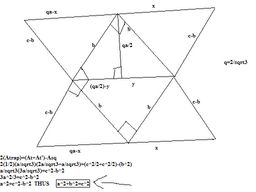# My Pythagorean Theorem proof

### Welcome to our community

#### iooqxpooi

##### Member
My Pythagorean Theorem proofRemember that (z-s)+s=z, that might help.

Ok, I just realized that you can't see the math...

Well, the box in the middle is a square, with side b. The large triangles have side c and are isosceles. The trapezoids have bases 2a/sqrt3 and a/sqrt3 with height a/sqrt3. With 2 congruent trapezoids. Thus...

2(Atrap)=(Atr+Atr')-Asq
2(1/2)(a/sqrt3)(2a/sqrt3+a/sqrt3)=(c^2/2+c^2/2)-(b^2)
a/sqrt3(3a/sqrt3)=c^2-b^2
3a^2/3=c^2-b^2
a^2=c^2-b^2
a^2+b^2=c^2#### Cosmo

##### Senior Member
Re: My Pythagorean Theorem proof

You're one smart kid, IggyI'm a bit confused as to what this has to do with the theory of time travel. You might want to clue us in...

#### StarLord

##### Senior Member
Re: My Pythagorean Theorem proof

Nothing. But hey! it's another formula brought to you by Iggy & Pythagoras. What more did you want?

#### iooqxpooi

##### Member
Re: My Pythagorean Theorem proofThanks...There really is no forum for non-time travel yet sciency things, so I just put it here...Mostly because I sometimes have time travel theories and sometimes I don't...I just want to be able to find it all on one forum.#### sosuemetoo

##### Active Member
Re: My Pythagorean Theorem proof

Iggy,

OT, but just wanted you to know that I'm glad to see you back again. Missed ya!

#### CaryP

##### Senior Member
Re: My Pythagorean Theorem proof

Second that Susan. Good to see you posting iooqxpooi. I don't understand what your original post said at all. Not enough brain pan on my end.

Cary